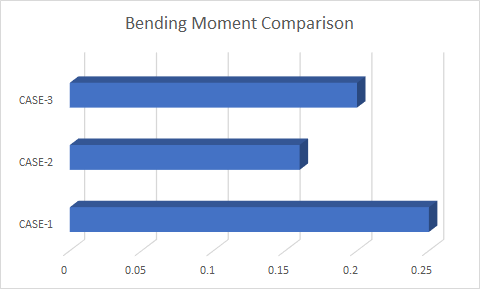### Bracing System - Structural arrangement that ensures stability in Longitudinal Direction

What happens to a building when it is subjected to wind loads? Any building or structures, in general, must ensure stability in two directions (Lateral & Longitudinal) to safely transfer loads from the location of application to the ground. Considering a typical steel warehouse building something similar to the following image, when it is subjected to wind load along the lateral direction, stability is ensured by the portal frame action. Lateral Direction - Along width of the building Longitudinal Direction - Along Length of the building The column and the rafter connected using a rigid joint act as a portal to sustain the lateral loads that act on the building. So, the building is fine in the lateral direction. What if the wind blows in the longitudinal direction?  How longitudinal force gets transferred through the system? In the longitudinal direction, when the force acts on the gable ends of the building, the first component to interact with the load is the cladding materials (

### How Load Position would affect the members bending capacity - "Bend the RULER"

Why it is easy to bend material in the middle rather than at the ends?

In order to understand the concept, one must clearly visualize the context.

I like to clarify two things here, firstly the bending capacity of a member. Secondly, the bending force applied.

For our easy understanding, Consider a 1m length ruler. Let's place it between the two tables and apply 1 kN load on the ruler. We are about to determine the bending capacity of the ruler as well as the bending moment developed due to the applied load.

BENDING CAPACITY:

It is nothing but the capacity or strength of the object to withstand the applied bending force.

The bending capacity is given by the simple bending equation as M/I = F/y

Where, M = Bending moment capacity of the section,

I = Moment of Inertia of the section

F = Yield strength of the material

y = Position of Neutral Axis

Investigating the above equation,

· The moment of inertia of the section depends upon its cross-sectional (width, depth & area of material) parameters.

· The yield strength of a material is a constant value for a particular material.

· The neutral axis depth for a symmetrical section will be at its half depth, considering the major axis.

From the above statements, we infer that whatever the length of the member, unless and until if the cross-section and the material don't vary, the moment capacity of the section remains the same.

So, it has nothing to do with the bending of the member.

BENDING MOMENT:

Let us consider 3 different cases for the determination of bending moment.

1. The load (1 kN) placed exactly at the center of the ruler.

2. The load (1 kN) placed 0.8m from one support (i.e. near one end of the ruler).

3. Let's move the tables close to each other, making the length of the ruler 0.8m and placing the load at the center.

CASE-1:

Length of the ruler = 1m; Load = 1 kN located at center

Bending Moment under the load = WL/4 = 1*1/4 = 0.25 kN.m

CASE-2:

Length of the ruler = 1m; Load = 1 kN located at 0.8m from one end.

Bending Moment under the load = W.a.b/L = 1*0.8*0.2/1 = 0.16 kN.m

CASE-3:

Length of the ruler = 0.8m; Load = 1 kN located at center

Bending Moment under the load = WL/4 = 1*0.8/4 = 0.2 kN.mWhat do we infer from this?

Comparing case-1 and case-2: The ruler is likely to bend more when the load is applied in the middle rather at the ends, because, the load acting at the middle develops a larger bending moment.

Comparing case-1 and case-3: As the length of the ruler decreases, even though the load acts in the middle, the moment value reduces for the shorter ruler. So, if we bend two members of the same material and different lengths, the lengthy member will bend more. With an increase in the length of the member, the bending moment also increases.